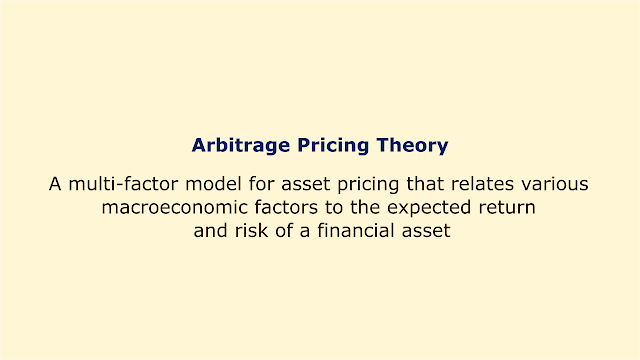# Arbitrage Pricing Theory (APT)Image: Moneybestpal.com

### Arbitrage Pricing Theory (APT) is a multi-factor model for asset pricing that relates various macroeconomic factors to the expected return and risk of a financial asset. It was proposed by economist Stephen Ross in 1976 as an alternative to the Capital Asset Pricing Model (CAPM), which only considers one factor, the market risk.

According to APT, an asset's expected return can be expressed as a linear function of its sensitivity to different risk factors and the risk premium associated with each factor. The formula for APT is:

E(R_i) = E(R_z) + (E(I_1) - E(R_z)) * β_1 + (E(I_2) - E(R_z)) * β_2 + ... + (E(I_n) - E(R_z)) * β_n

where:
• E(R_i) is the expected return on asset i
• E(R_z) is the risk-free rate of return
• E(I_j) is the expected return on factor j
• β_j is the sensitivity of asset i to factor j
• n is the number of factors

The risk factors in APT include macroeconomic factors like inflation, GDP growth, interest rates, exchange rates, etc. that have an impact on the returns of all market assets. The number and choices of factors are flexible and based on both the analyst's expertise and empirical data. The majority of the volatility in asset returns, however, can typically be explained by four or five factors.

According to APT, there are possibilities for arbitrage in the market, which allows investors to benefit without taking any risks by taking advantage of any differences between an asset's fair value and its actual worth by investing in it when it is cheap and selling it when it is overpriced. Eventually, this procedure will correct any mispricing and return the asset price to its equilibrium level.

APT is a more flexible and complex model than CAPM, as it allows for multiple sources of risk and does not require any assumptions about market efficiency or investor preferences. However, it also has some limitations, such as:
• The risk variables are not well identified or quantified.
• The method for calculating the risk premiums for each factor is not specified.
• It excludes any unsystematic risk particular to a sector or an asset.
• The relationships between the risk variables are not taken into account.

APT is a helpful technique for value investing portfolio analysis since it can help uncover assets that are briefly mispriced and offer enticing rewards in relation to their risks.
Tags